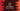# Java Program to find the first digit of a positive or negative number## Java Program to find the first digit of a positive or negative number:

This post will show you how to find the first digit of a positive or negative number in Java.

The easiest way to solve this is by keep dividing the by 10 until it become less than 10 or one digit. That will be the first digit of the number. For example, for 987,

``````987 / 10 = 98
98 / 10 = 9``````

First, we divide 987 by 10. It become 98. Then we divide it by 10 again and it become 9, which is the first digit of 987.

### Method 1: By diving the number repeatedly :

In this method, we are using a while loop. This loop keeps dividing the number by 10 repeatedly until it become less than 9 or one digit. Also, we are taking the absolute value of the entered number because we are doing it for both positive and negative values.

Taking an absolute value makes it always positive.

``````import java.util.Scanner;

import static java.lang.Math.abs;

public class Main {
public static void main(String[] args) {
Scanner sc = new Scanner(System.in);

System.out.println("Enter a number : ");
int no = sc.nextInt();

int firstDigit = abs(no);

while(firstDigit > 9){
firstDigit = firstDigit/10;
}
System.out.println("First digit of the number : " + firstDigit);
}
}``````

This program will give output as like below:

``````Enter a number :
-88787
First digit of the number : 8

Enter a number :
7639373
First digit of the number : 7``````

### Method 2: By converting the integer to string:

We can convert the integer to string using toString and pick the character at index 0, i.e. the first digit of the integer. We can again convert that character to an integer by using Integer.parseInt.

Below is the complete program:

``````import java.util.Scanner;

import static java.lang.Math.abs;

public class Main {
public static void main(String[] args) {
Scanner sc = new Scanner(System.in);

System.out.println("Enter a number : ");
int no = sc.nextInt();

System.out.println("First digit of the number : " + Integer.parseInt("" + Integer.toString(abs(no)).charAt(0)));
}
}``````

### Method 3:

Another way to get the first digit is by converting the integer to a string and picking the first character by using substring method. We can convert the character to integer using Integer.parseInt(). Below is the complete program:

``````import java.util.Scanner;

import static java.lang.Math.abs;

public class Main {
public static void main(String[] args) {
Scanner sc = new Scanner(System.in);

System.out.println("Enter a number : ");
int no = sc.nextInt();

System.out.println("First digit of the number : " + Integer.parseInt(Integer.toString(abs(no)).substring(0, 1)));
}
}``````

All of these programs will give similar output.